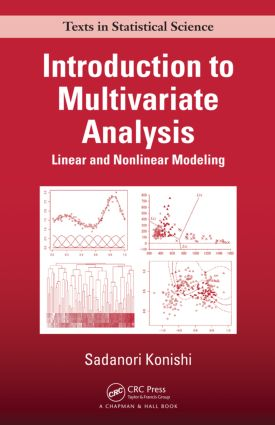# Introduction to Multivariate Analysis

## Linear and Nonlinear Modeling, 1st Edition

Chapman and Hall/CRC

338 pages | 80 B/W Illus.

##### Purchasing Options:\$ = USD
Hardback: 9781466567283
pub: 2014-06-06
\$110.00
x
eBook (VitalSource) : 9780429099267
pub: 2014-06-06
from \$28.98

FREE Standard Shipping!

### Description

Select the Optimal Model for Interpreting Multivariate Data

Introduction to Multivariate Analysis: Linear and Nonlinear Modeling shows how multivariate analysis is widely used for extracting useful information and patterns from multivariate data and for understanding the structure of random phenomena. Along with the basic concepts of various procedures in traditional multivariate analysis, the book covers nonlinear techniques for clarifying phenomena behind observed multivariate data. It primarily focuses on regression modeling, classification and discrimination, dimension reduction, and clustering.

The text thoroughly explains the concepts and derivations of the AIC, BIC, and related criteria and includes a wide range of practical examples of model selection and evaluation criteria. To estimate and evaluate models with a large number of predictor variables, the author presents regularization methods, including the L1 norm regularization that gives simultaneous model estimation and variable selection.

For advanced undergraduate and graduate students in statistical science, this text provides a systematic description of both traditional and newer techniques in multivariate analysis and machine learning. It also introduces linear and nonlinear statistical modeling for researchers and practitioners in industrial and systems engineering, information science, life science, and other areas.

### Reviews

"The presentation is always clear and several examples and figures facilitate an easy understanding of all the techniques. The book can be used as a textbook in advanced undergraduate courses in multivariate analysis, and can represent a valuable reference manual for biologists and engineers working with multivariate datasets."

—Fabio Rapallo, Zentralblatt MATH 1296

"This is an excellent textbook for upper-class undergraduate and graduate level students. The prerequisites are an introductory probability and statistics and linear algebra courses. To aid the student in the understanding and use of vector and matrix notations, and to emphasize that importance, the author appropriately uses the algebraic notation accompanied by the vector and matrix notations when needed; additionally, the accompanying geometrical interpretation are presented in clear diagrams. The writing style is crisp and clear. A pleasant format that the author used is to summarily review relevant topics in a narrative style to pave the way into a new topic. The textbook is accessible to students and researchers in the social sciences, econometrics, biomedical, computer and data science fields. This is the kind of textbook that a student or professional researcher will consult many times."

—Stephen Hyatt, International Technological University

Introduction

Regression Modeling

Classification and Discrimination

Dimension Reduction

Clustering

Linear Regression Models

Relationship between Two Variables

Relationships Involving Multiple Variables

Regularization

Nonlinear Regression Models

Modeling Phenomena

Modeling by Basis Functions

Basis Expansions

Regularization

Logistic Regression Models

Risk Prediction Models

Multiple Risk Factor Models

Nonlinear Logistic Regression Models

Model Evaluation and Selection

Criteria Based on Prediction Errors

Information Criteria

Bayesian Model Evaluation Criterion

Discriminant Analysis

Fisher’s Linear Discriminant Analysis

Classification Based on Mahalanobis Distance

Variable Selection

Canonical Discriminant Analysis

Bayesian Classification

Bayes’ Theorem

Classification with Gaussian Distributions

Logistic Regression for Classification

Support Vector Machines

Separating Hyperplane

Linearly Nonseparable Case

From Linear to Nonlinear

Principal Component Analysis

Principal Components

Image Compression and Decompression

Singular Value Decomposition

Kernel Principal Component Analysis

Clustering

Hierarchical Clustering

Nonhierarchical Clustering

Mixture Models for Clustering

Appendix A: Bootstrap Methods

Appendix B: Lagrange Multipliers

Appendix C: EM Algorithm

Bibliography

Index

### Subject Categories

##### BISAC Subject Codes/Headings:
MAT029000
MATHEMATICS / Probability & Statistics / General